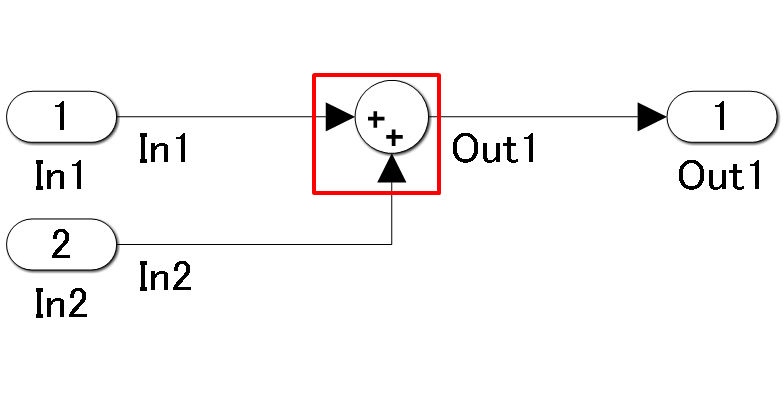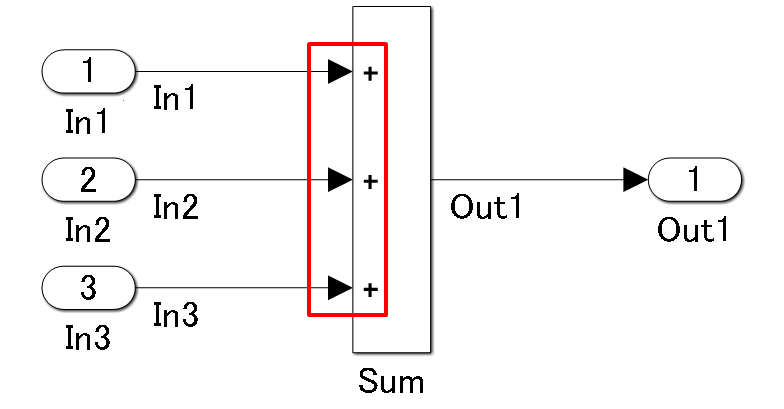jc_0121: Usage of add and subtraction blocks

Sub ID Recommendations

• NA-MAAB — a

• JMAAB — a, b, c

All

Rule

Sub ID a

The icon shape of the add and subtraction Sum block shall be rectangular.

When used in a feedback loop, the icon shape can be round.

Custom Parameter

Not Applicable

Example — Correct

The icon shape of the add and subtraction Sum block shall be rectangular.The second input to the add and subtraction Sum block is a feedback loop, so the icon shape is round.Example — Incorrect

This is not a feedback loop, but the icon shape of the add and subtractionSum block is round.Sub ID b

The + mark shall be used for the first input to the add and subtraction Sum block.

For a feedback loop, the first input can be set by using the - mark.

Custom Parameter

Not Applicable

Example — Correct

The + mark is used for the first input to the add and subtraction Sum block.The second input to the add and subtraction Sum block is a feedback loop, so the - mark is usedExample — Incorrect

The sign for the first input to the add and subtraction Sum block is the - mark.Sub ID c

The add and subtraction Sum block shall not have more than two inputs.

Custom Parameter

Not Applicable

Example — CorrectExample — Incorrect

The add and subtraction Sum block has three inputs.Rationale

Sub ID a:

Sub ID b:

• Readability of the control specification improves when the sign for the first input is consistent.

Sub ID c:

• The order of operations is clearly defined.